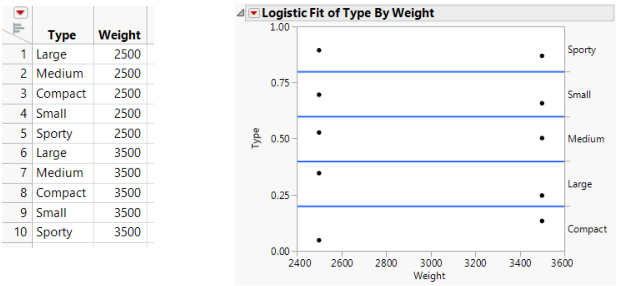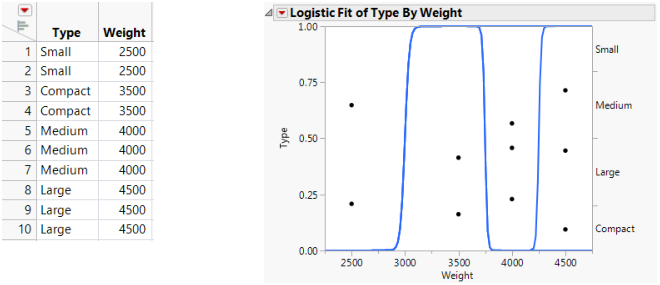Publication date: 08/13/2020

## Additional Example of a Logistic Plot

This example uses the Car Physical Data.jmp sample data table to show an additional example of a logistic plot. Suppose you want to use weight to predict car size (Type) for 116 cars. Car size can be one of the following, from smallest to largest: Sporty, Small, Compact, Medium, or Large.

1. Select Help > Sample Data Library and open Car Physical Data.jmp.

2. In the Columns panel, right-click the icon to the left of Type, and select Ordinal.

3. Right-click Type and select Column Info.

4. From the Column Properties menu, select Value Order.

5. Verify that the data are in the following top-down order: Sporty, Small, Compact, Medium, Large.

6. Click OK.

7. Select Analyze > Fit Y by X.

8. Select Type and click Y, Response.

9. Select Weight and click X, Factor.

10. Click OK.

The report window appears.

Figure 8.6 Example of Type by Weight Logistic PlotNote the following observations:

The first (bottom) curve represents the probability that a car at a given weight is Sporty.

The second curve represents the probability that a car is Small or Sporty. Looking only at the distance between the first and second curves corresponds to the probability of being Small.

As you might expect, heavier cars are more likely to be Large.

Markers for the data are drawn at their x-coordinate. The y position is jittered randomly within the range corresponding to the response category for that row.

If the x -variable has no effect on the response, then the fitted lines are horizontal and the probabilities are constant for each response across the continuous factor range. Figure 8.7 shows a logistic plot where Weight is not useful for predicting Type.

Figure 8.7 Examples of Sample Data Table and Logistic Plot Showing No y by x RelationshipNote: To re-create the plots in Figure 8.7 and Figure 8.8, you must first create the data tables shown here, and then perform steps 7-10 at the beginning of this section.

If the response is completely predicted by the value of the factor, then the logistic curves are effectively vertical. The prediction of a response is near certain (the probability is almost 1) at each of the factor levels. Figure 8.8 shows a logistic plot where Weight almost perfectly predicts Type.

Figure 8.8 Examples of Sample Data Table and Logistic Plot Showing an Almost Perfect y by x RelationshipIn this case, the parameter estimates become very large and are labeled unstable in the regression report. In these cases, you might consider using the Generalized Linear Model personality with Firth bias-adjusted estimates. See Launch the Generalized Linear Model Personality in Fitting Linear Models.